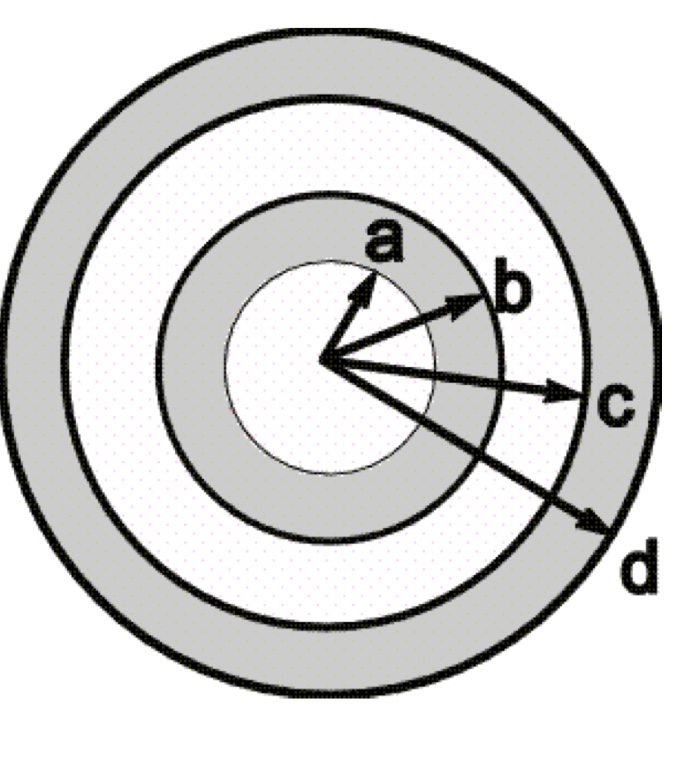# Problem: A small conducting spherical shell with inner radius a and outer radius b is concentric with a larger conducting spherical shell with inner radius c and outer radius d. The inner shell has total charge +3q, and the outer shell has charge +5q. (a) Calculate the magnitude and direction of electric field E in terms of q and the distance r from the common center of the two shells for (i) r &lt; a;(ii) a &lt; r &lt; b;(iii) b &lt; r &lt; c;(iv) c &lt; r &lt; d;(v) r &gt; d.(b) What is the total charge on the (i) inner surface of the small shell; (ii) outer surface of the small shell; (iii) inner surface of the large shell; (iv) outer surface of the large shell?

###### FREE Expert Solution

In this problem, we'll be dealing with electric fields and a conducting sphere.

Gauss law:

$\overline{){{\mathbf{\varphi }}}_{{\mathbf{E}}}{\mathbf{=}}{\mathbf{\oint }}{\mathbf{E}}{\mathbf{·}}{\mathbf{d}}{\mathbf{A}}{\mathbf{=}}\frac{\mathbf{Q}}{{\mathbf{\epsilon }}_{\mathbf{0}}}}$

(a)

(i) The charge enclosed at the center of the sphere is zero.

98% (201 ratings)###### Problem Details

A small conducting spherical shell with inner radius a and outer radius b is concentric with a larger conducting spherical shell with inner radius c and outer radius d. The inner shell has total charge +3q, and the outer shell has charge +5q.

(a) Calculate the magnitude and direction of electric field E in terms of q and the distance r from the common center of the two shells for

(i) r < a;
(ii) a < r < b;
(iii) b < r < c;
(iv) c < r < d;
(v) r > d.

(b) What is the total charge on the (i) inner surface of the small shell; (ii) outer surface of the small shell; (iii) inner surface of the large shell; (iv) outer surface of the large shell?Frequently Asked Questions

What scientific concept do you need to know in order to solve this problem?

Our tutors have indicated that to solve this problem you will need to apply the Electric Fields with Calculus concept. You can view video lessons to learn Electric Fields with Calculus. Or if you need more Electric Fields with Calculus practice, you can also practice Electric Fields with Calculus practice problems.